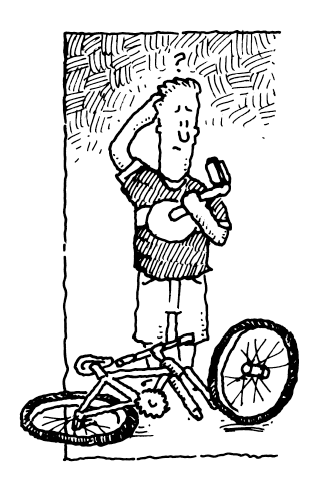### Home > PC > Chapter 11 > Lesson 11.2.2 > Problem11-90

11-90.The pedal shaft on a standard bicycle is about $7$ inches long, and the center of the bottom bracket to which the pedal shaft is attached is about $11$ inches above the ground. Sketch a graph that shows the relationship of the angle of the shaft (for one pedal) in standard position (as if the point of attachment were the origin of a set of $x$-$y$axes shifted up $11$ units) to the height of the pedal above the ground as a rider pedals the bike. Assume the pedal starts in its lowest position and takes $2$ seconds to make one complete rotation.

1. Write an equation for a function that represents your graph.

Sketch the situation. Identify the amplitude, period, and any shifting.

2. Jack feels that the best position for the pedal to start riding is when the pedal is at $10$ inches and heading downward. What is the first time the pedal will be in this position?

Set your equation from part (a) equal to $10$ and solve.

Use the eTool below to see a sample animation.
Click the link to the right for full version. 11-90 HW eTool